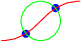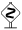# PyX — Example: path/intersect.py

0.4 KB
7.8 KB
0.8 KB
1.4 KB
0.7 KB

## Intersection points between paths```from pyx import *

p1 = path.curve(0, 0, 1, 0, 1, 1, 2, 1)
p2 = path.circle(1, 0.5, 0.5)

(a1, a2), (b1, b2) = p1.intersect(p2)

x1, y1 = p1.at(a1)
x2, y2 = p1.at(a2)

c = canvas.canvas()
c.fill(path.circle(x1, y1, 0.1), [color.rgb.blue])
c.fill(path.circle(x2, y2, 0.1), [color.rgb.blue])
c.stroke(p1, [color.rgb.red])
c.stroke(p2, [color.rgb.green])
c.writeEPSfile("intersect")
c.writePDFfile("intersect")
c.writeSVGfile("intersect")
```

### Description

The `intersect` method of a path allows for the calculation of intersection points between this path and the second path passed to the `intersect` method. The return value of the intersect method is a tuple of two lists, where each list contains parametrization instances for the intersection points. The first list are the parameters for the path the `intersect` method was called for. The second list are the parameter values for the path passed to the `intersect` method. Thus we can calculate the first intersection point `x1, y1` by

```x1, y1 = p1.at(a1)
```

as done in the example or alternatively by

```x1, y1 = p2.at(b1)
```When several intersections between two paths occur, the order of the intersection points is defined by the order in which the points are passed when walking along the first path.